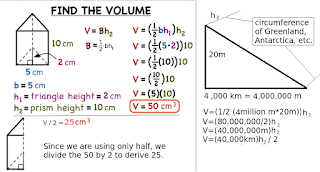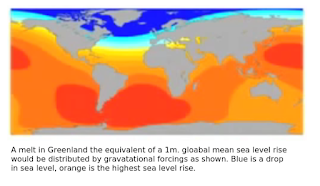Saturday, January 16, 2016

The Gravity of Sea Level Change - 3Fig. 1 Vast amounts of water held by ice sheet gravity
In this post I want to talk about a new source of sea level change (SLC) that takes place without any additional ice sheet melt.

I want to write about the SLC which takes place as gravity-held water along the coasts of ice sheet covered land masses is released as the ice sheet's force of gravity decreases.

I am talking about the amount of sea water that is already there in the sea when the ice sheet melts or calves.Fig. 2 Calculating the volume of water in the wedge

I am talking about sea water that is not the same as the water contained in the ice sheet, which will melt later to become additional sea water.

Notice the difference as shown on the right side of Fig. 1, where the ice sheet is the white mass on top of the dark grey land mass, and where the sea water held by gravity is a blue wedge shape touching the dark grey coastline of the dark grey land mass.

In the search for a reason that SLC software underestimates observed values, I want to attempt to calculate the amount of sea level fall (SLF) of water (deep blue color hugging the coast of Antarctica in Fig. 1) which, during SLC, is relocated to a sea level rise (SLR) area of the oceans (shown as the cyan, orange, and red colors in Fig. 1; Greenland SLF / SLC is shown in Fig. 3).

In other words, when water drops in one place, it rises in another place because the volume of sea water is not changed in this scenario, it is just rearranged.Fig. 3
We can use the formula for the volume of a prism shape, then divide by 2 (halve it) to derive the volume of a wedge-shaped triangle, which is mathematically equal to the volume of water in the wedge.

On the right side of Fig. 2 are some of the actual dimensions: 1) 20 meters as the height of the water (marked "h1" on Fig. 1 and Fig. 2); 2) next, marked as "h2" is the length of the prism (the length of the coastline the gravity-held water is pulled up against), and 3) the base marked as "b" is the full length of the prism base, (in today's application it is 2,000 km, which we eventially divide by 2; it is the distance from h1 to the "hinge line" which is h2).

The prism volume formula works like this:
V = (1/2 (b h1)) h2
where:
b = 4,000 km (4,000,000 m)
h1 = 20 m (Mitrovica suggest figure in video below)
h2 = length of relevant coastline
thus:

V = (1/2 (4,000,000 m * 20 m)) h2
V = (1/2 (80,000,000 m)) h2
V = (80,000,000 m / 2) h2
V = 40,000,000 m * h2
V = 40,000 km * h2

to apply the calculation to Greenland:
Greenland coastline (h2) = 44,087 km
(link to source of coastline length)

thus:
V = 40,000 km * 44,087 km
V = 1,763,480,000 km3

to adapt it to the water wedge,
divide by 2 (wedge is 1/2 of prism)

thus:
V = 1,763,480,000 km3 / 2
V = 881,740,000 km3 of sea water relocated from Greenland

to apply the calculation to Antarctica:
Antarctica coastline (h2) = 17,968 km
V = 40,000 km * 17,968 km
V = 718,720,000 km3 / 2
V = 359,360,000 km3 of sea water relocated from Antarctica

to sum both of them:
V = 881,740,000 km3 + 359,360,000 km3
V = 1,241,100,000 km3 total sea water relocated from both
That is the cubic volume of water that will be released and relocated as a result of loss of ice sheet gravity due to ice melt sufficient to cause 1m of global average SLR by each location.

Which is calculated to cause 20 meters of SLF around Greenland and/or Antarctica, then, when relocated will cause 1 meter of SLR globally for each of them, 2m total (The Gravity of Sea Level Change, 2).

I have been wondering if the SLC projection models take this into consideration, seeing as how they have consistently underestimated SLR:
"Why doesn't it add up?

Together, thermal expansion and melting snow and ice contributed 2.8 ± 0.7 mm per year to sea level rise between 1993 and 2003 (Bindoff et al., 2007). However, that total was lower than the actual observed global mean sea level rise for period by about 10%. What is causing the rest of sea level rise? This discrepancy is often referred to as the sea level enigma, and scientists have offered a number of possible explanations." - Weather Underground

"Expect more water to lap at your shores. That’s the take-home message from two studies out this week that look at the latest data on sea level rise due to climate change.

The first shows that current projections for the end of the century may seriously underestimate the rise in global sea levels. The other, on the ice sheets of Greenland and Antarctica, looks at just how much of the water stored up there has been moving into the oceans." Projections of sea level rise are vast underestimates
Gravity is an import factor that should be included in models so as to add some useful and essential figures.

Watch the video below, if you haven't already, to acquire some additional SLC prowess.

The next post in this series is here, the previous post in this series is here.

A discussion of, among other things, SLC as impacted by ice sheet mass and gravity: